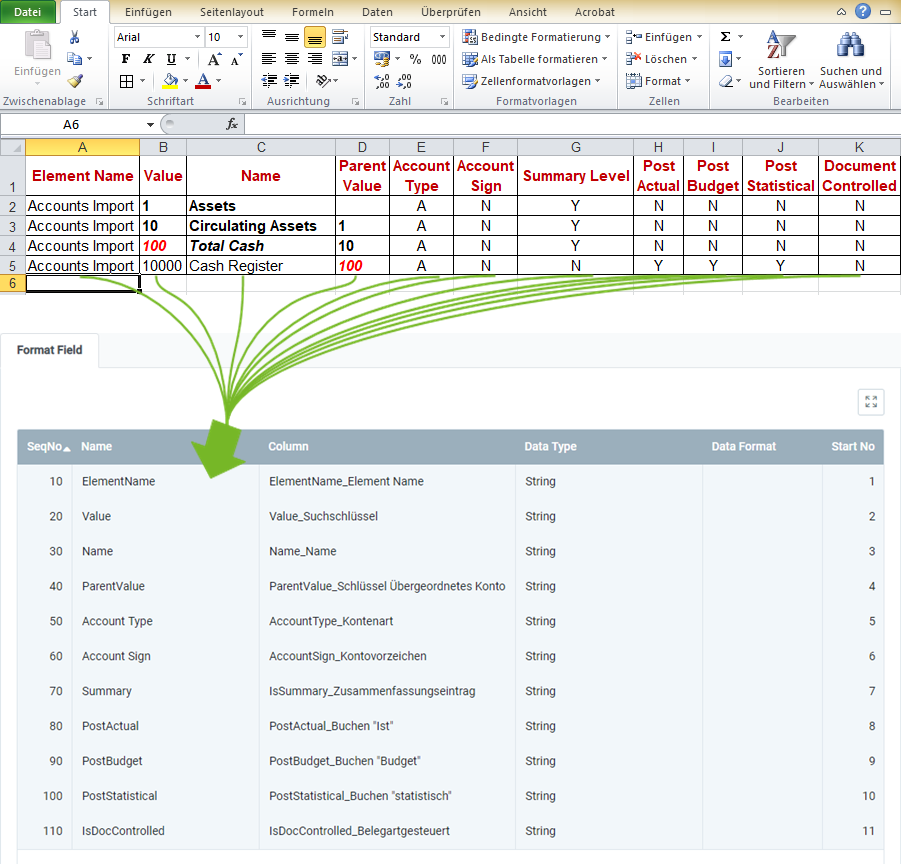Wonach suchst Du?
What are you looking for?

# Format example for importing chart of accounts data

## Overview

For importing chart of accounts data, you require an import format that is configured with the database table Import Account.

The example below compares the data content of a file from a spreadsheet software (here, e.g., an Excel file prior to its conversion to a CSV or TXT file) with the import format for chart of accounts data:### Explanatory Notes to the Example

• Column A of the Excel spreadsheet (Element Name) is in the first place, ergo the corresponding format field is given the Start No. 1. Consequently, the format field for Column B receives the Start No. 2 etc.
The order (SeqNo) of the format fields is therefore irrelevant.

Note: metasfresh does not expect any column names in the import file. Simply the position of the column must correspond to the start number.

• The Name of the format field may be chosen freely and does not have to coincide with the column name in the import file.
• The Column of the format field specifies where metasfresh shall transfer the content of the column from the import file.
• The Data Type specifies whether the type of the import data is, e.g., a String or a Number.

### Some useful notes

The mandatory fields are essential for a successful data import!

Mandatory Field Field Name Example Note
X Element Name Accounts Import Name of the chart of accounts
X Value 10 Search key of the accounting element (is also used to structure the elements among each other).
X Name Circulating Assets Name of the accounting element
Parent Value 1 Search key of the parent (summary) account (is also used to structure the elements among each other).
Account Type • A = Assets
• E = Expense
• O = Owner’s Equity
• R = Revenue
• M = Memo
• L = Liabilities
Type of the account
Account Sign • N = Natural
• C = Credit
• D = Debit
Indicates the natural sign of the account as a debit or credit.
Summary Level • Y / true = Yes
• N / false = No
In a tree structure, summary entities represent a superior branch that combines child entries and can therefore be used for reporting.
Post Actual • Y / true = Yes
• N / false = No
Indicates whether actual values can be posted to an element.
Post Budget • Y / true = Yes
• N / false = No
Indicates whether budget values can be posted to an element.
Post Statistical • Y / true = Yes
• N / false = No
Indicates whether statistical values can be posted to an element.
Document Controlled • Y / true = Yes
• N / false = No
If an account is controlled by a document, you cannot post to it manually.

## Next Steps

View source file on GitHub.com